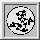Up: Qhull manual: contents
Up: ProgramsOptionsOutputFormatsGeomviewPrintQhullPrecisionTraceFunctions (local)
Up: Qhull code
To: GeomGlobalIoMemMergePolyQhullSetStatUser

## poly_r.c, poly2_r.c -- polyhedron operations

Qhull uses dimension-free terminology. Qhull builds a polyhedron in dimension d. A polyhedron is a simplicial complex of faces with geometric information for the top and bottom-level faces. A (d-1)-face is a facet, a (d-2)-face is a ridge, and a 0-face is a vertex. For example in 3-d, a facet is a polygon and a ridge is an edge. A facet is built from a ridge (the base) and a vertex (the apex). See Qhull's data structures.

Qhull's primary data structure is a polyhedron. A polyhedron is a list of facets. Each facet has a set of neighboring facets and a set of vertices. Each facet has a hyperplane. For example, a tetrahedron has four facets. If its vertices are a, b, c, d, and its facets are 1, 2, 3, 4, the tetrahedron is

• facet 1
• vertices: b c d
• neighbors: 2 3 4
• facet 2
• vertices: a c d
• neighbors: 1 3 4
• facet 3
• vertices: a b d
• neighbors: 1 2 4
• facet 4
• vertices: a b c
• neighbors: 1 2 3

A facet may be simplicial or non-simplicial. In 3-d, a simplicial facet has three vertices and three neighbors. A nonsimplicial facet has more than three vertices and more than three neighbors. A nonsimplicial facet has a set of ridges and a centrum.

A simplicial facet has an orientation. An orientation is either top or bottom. The flag, facet->toporient, defines the orientation of the facet's vertices. For example in 3-d, 'top' is left-handed orientation (i.e., the vertex order follows the direction of the left-hand fingers when the thumb is pointing away from the center). Except for axis-parallel facets in 5-d and higher, topological orientation determines the geometric orientation of the facet's hyperplane.

A nonsimplicial facet is due to merging two or more facets. The facet's ridge set determine a simplicial decomposition of the facet. Each ridge is a 1-face (i.e., it has two vertices and two neighboring facets). The orientation of a ridge is determined by the order of the neighboring facets. The flag, facet->toporient,is ignored.

A nonsimplicial facet has a centrum for testing convexity. A centrum is a point on the facet's hyperplane that is near the center of the facet. Except for large facets, it is the arithmetic average of the facet's vertices.

A nonsimplicial facet is an approximation that is defined by offsets from the facet's hyperplane. When Qhull finishes, the outer plane is above all points while the inner plane is below the facet's vertices. This guarantees that any exact convex hull passes between the inner and outer planes. The outer plane is defined by facet->maxoutside while the inner plane is computed from the facet's vertices.

Qhull 3.1 includes triangulation of non-simplicial facets ('Qt'). These facets, called tricoplanar, share the same normal. centrum, and Voronoi center. One facet (keepcentrum) owns these data structures. While tricoplanar facets are more accurate than the simplicial facets from joggled input, they may have zero area or flipped orientation.

» Geom GlobalIoMemMergePolyQhullSetStatUser

### »poly_r.h constants

• DUPLICATEridge special value for facet->neighbor to indicate a duplicate ridge
• MERGEridge special value for facet->neighbor to indicate a merged ridge

### »Indexed FOREACH macros

• FOREACHfacet_i_ assign 'facet' and 'facet_i' to each facet in facet set
• FOREACHneighbor_i_ assign 'neighbor' and 'neighbor_i' to each facet in facet->neighbors or vertex->neighbors
• FOREACHpoint_i_ assign 'point' and 'point_i' to each point in points set
• FOREACHridge_i_ assign 'ridge' and 'ridge_i' to each ridge in ridges set
• FOREACHvertex_i_ assign 'vertex' and 'vertex_i' to each vertex in vertices set
• FOREACHvertexreverse12_ assign 'vertex' to each vertex in vertex set; reverse the order of first two vertices

### »Other macros for polyhedrons

• getid_ return ID for a facet, ridge, or vertex
• otherfacet_ return neighboring facet for a ridge in a facet

### »Check functionsThe Geometry Center Home Page